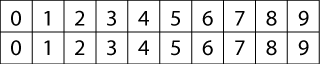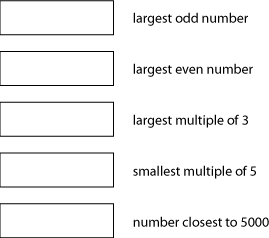#### You may also like### Chocolate

There are three tables in a room with blocks of chocolate on each. Where would be the best place for each child in the class to sit if they came in one at a time?### Four Triangles Puzzle

Cut four triangles from a square as shown in the picture. How many different shapes can you make by fitting the four triangles back together?### Cut it Out

Can you dissect an equilateral triangle into 6 smaller ones? What number of smaller equilateral triangles is it NOT possible to dissect a larger equilateral triangle into?

# Four-digit Targets

## Four-digit Targets

Four-digit Targets printable sheet

You have two sets of the digits from $0$ to $9$.The idea is to arrange these digits in the five boxes to make four-digit numbers as close to the target number as possible.
You may use each digit once only.This activity has been adapted from one of BEAM's free Maths of the Month resources, which unfortunately are no longer available. You can play it as a game against a friend using this sheet from BEAM.

### Why do this problem?

This problem would fit in well when members of the group are partitioning, rounding and ordering four-digit whole numbers. It requires considerable understanding of how the number system works. Playing the game, and discussing it afterwards, can really help to develop a firm concept of place value.

### Possible approach

You could start by writing a four-digit number on the board, for example $6149$, and asking how the digits could be rearranged to make the numbers that are the largest, smallest and nearest to $5000$. Could they be rearranged to make a multiple of $5$? Of $3$? If not, why not?

Next you could introduce an item in the problem such as making the largest possible even number using only two of each digit. Alternatively, you could make up your own examples such as the smallest even number or the nearest to $7000$. You will need to establish whether $0$ can be used at the beginning of a number. This, in itself, can form an interesting discussion point. (The final decision itself does not matter - it is the reasons that are important, and the fact that the children feel as if it is their decision!)

Probably the best way of continuing on this problem is to use this sheet from BEAM and for learners to work competitively in pairs. It is ideal if each player can also have two sets of digit cards to use to make the numbers.
Once learners have played a few times, instigate a general discussion on the best strategies and the nearest that anyone got to the target. You could repeat what you did at the start and give learners numbers such as $2681$ and ask them how they would rearrange this to make the highest/lowest number possible and why this is so. This will make a good assessment opportunity.

### Key questions

Why have you put a $5$ here?

Where is the best place to put $9$ when you are aiming for the lowest/highest number?

### Possible extension

Learners could make up their own criteria for a new game possibly using four five-digit numbers.

### Possible support

Children could use this simpler version of the problem.